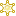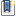File : Details

# Decimal to fraction

Changes a decimal number to a fraction
Details
Version
1.0
Author
Maintainer
Category
Supported Scilab Version
5.3
Creation Date
March 19, 2011Description
```            Changes a decimal number to a fraction within a given accuracy

Examples:

dec2frac(%pi,.1)
num = 22
den = 7

dec2frac(%pi,.001)
num = 106
den = 333

dec2frac(11/19,.001)
num = 11
den = 19

```Files (0)News (0)Comments (5)Comment from Samuel Gougeon -- March 22, 2011, 09:30:33 PM
```Hello David,
This function already exists in Scilab: Have a look at rat()
Regards
Samuel
```Comment from david adams-- March 22, 2011, 09:36:44 PM
```1.  If I cannot find a function immediately, then it might as well not exist.  This is one
of the problems with Scilab documentation.

For example, the following search terms do not get the proper function:
dec
frac
dec2frac

When I type if 'rat' the response is 'Floating point rational approximation' which is not
a useful description of what I am looking for.  I want to convert a decimal number to a
fraction.

2.  Who said posts in the file exchange have to complement functions in Scilab ?!?

```Comment from Nelson Lombardo -- October 4, 2012, 10:49:15 PM
```Great work!
Regards
Nelson```Comment from Felipe Santos -- October 22, 2015, 07:52:21 PM
```Hi there,

I would like to make a check the accuracy on the number of the decimals after the comma,
in a matriz.

For example I will divide 1 number by another and the result will approximate to 0, it
will be like 0.999999934757613

I want the calculation check to be as accurate as 9 points after the comma, so in my
calculation the program will check while x <= 0.0000000001 ...run the following code...

ANd it will stop when the accuracy reach 9 digits close to 1.

Is there a command like this in scilab, i remember at R, this command is epsolon i
believe...

thank you!```Comment from Wander Stabenow -- March 2, 2016, 04:04:05 AM
```I'm new to SciLab and using it at home to do Linear Algebra homework. In the classroom
we are using MatLab which can be configured to show decimal values in fraction format
via command "format rat". Since I couldn't find a way to do the same in SciLab I
was
looking for a way to decimals converted to fraction in SciLab.

Where can I get this "dec2frac" function that David Adams posted here? (I was
lookingLeave a comment
Login withEmail notifications